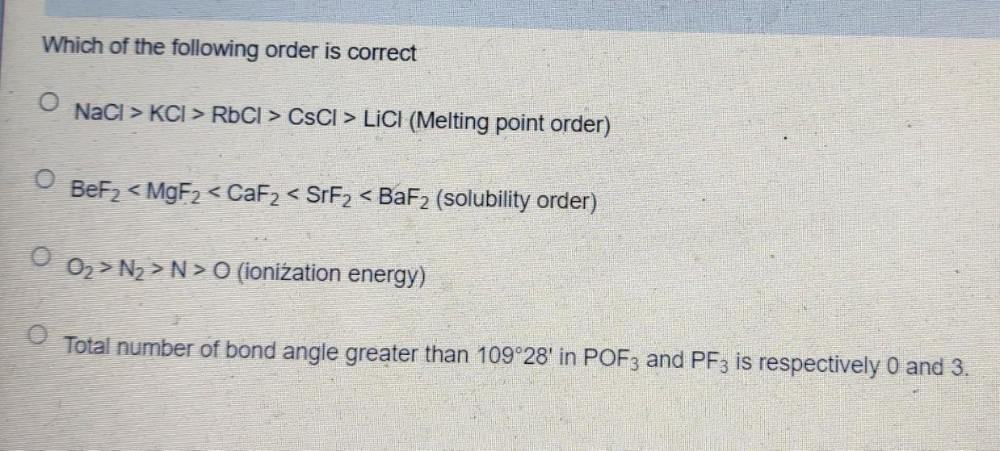Question:

# Which of the following order is correct O NaCl > KCl > RbCl > CsCl > LiCl (Melting point order) ○ BeF₂ < MgF₂ < CaF₂ < SrF₂ < BaF₂ (solubility order) O O₂ > N₂ > N > O (ionization energy) O Total numbWhich of the following order is correct O NaCl > KCl > RbCl > CsCl > LiCl (Melting point order) ○ BeF₂ < MgF₂ < CaF₂ < SrF₂ < BaF₂ (solubility order) O O₂ > N₂ > N > O (ionization energy) O Total number of bond angle greater than 109°28' in POF3 and PF3 is respectively 0 and 3.